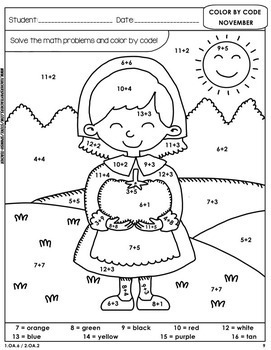# Color by Code: Math – NOVEMBER – 1st Grade - Common Core AlignedSubject
Resource Type
Format
Zip (16 MB|59 pages)
Standards
\$3.20
List Price:
\$4.00
You Save:
\$0.80
\$3.20
List Price:
\$4.00
You Save:
\$0.80

#### Also included in

1. Color by Code – MATH BUNDLE: A Full Year of Color by Codes!This Math bundle includes EVERY Math Puzzle from my Color by Code: Months collection!. Each sheet is seasonally-themed and makes the task of learning math facts a fun activity! These puzzles can be used as centers, bell ringers, homework,
\$96.00
\$160.00
Save \$64.00
2. Save money getting all my MATH - Color by Codes - 1st Grade in one single BUNDLE! :)
\$28.80
\$40.00
Save \$11.20

### Description

Color by Code: Math – NOVEMBER - 1st Grade - Common Core Aligned

With these cool puzzles your students will enjoy Math at the same time as they review the following Common Core Standards:

K.GA.2 / 1.OA.6 / 1.OA.8 / 2.OA.2

This pack includes 11 puzzles:

Puzzle 1

Puzzle 2

Puzzle 3

Determine the unknown number in an addition equation relating three whole numbers.

Puzzle 4

Determine the unknown number in a subtraction equation relating three whole numbers.

Puzzle 5

Version 1: Subtract within 20.

Version 2: Add and subtract within 20.

Puzzle 6

Subtract within 20.

Puzzle 7

Version 1: Determine the unknown number in an addition equation relating three whole numbers.

Version 2: Color by determining the unknown number in an addition or subtraction equation relating three whole numbers.

Puzzle 8

Puzzle 9

Version 2: Color by determining the unknown number in an addition or subtraction equation relating three whole numbers.

Puzzle 10

Puzzle 11

Correctly name shapes regardless of their orientations or overall size: square, rectangle, triangle, oval, circle, hexagon, rhombus.

I include the puzzles in black and white. I also include a full color version of each puzzle which you can use as the answer key. In order to suit everybody´s needs I also include the answer keys in black and white, and in color too. That makes a total of 59 pages. Please let me know if you have any specific need!

3 of the puzzles are for Thanksgiving. The rest are typical November scenes.

After the children have colored the puzzles, you can cut out the picture portion of each printable to decorate your classroom :)

*You can check my Pinterest Boards for more cool materials!

MATH – COMMON CORE

SPANISH TEACHER – EDUCATIVE MATERIALS

SUPERHEROES IN THE CLASSROOM!

FREE EDUCATIVE MATERIALS!

Total Pages
59 pages
Included
Teaching Duration
2 Weeks
Report this Resource to TpT
Reported resources will be reviewed by our team. Report this resource to let us know if this resource violates TpT’s content guidelines.

### Standards

to see state-specific standards (only available in the US).
Correctly name shapes regardless of their orientations or overall size.
Fluently add and subtract within 20 using mental strategies. By end of Grade 2, know from memory all sums of two one-digit numbers.
Determine the unknown whole number in an addition or subtraction equation relating three whole numbers. For example, determine the unknown number that makes the equation true in each of the equations 8 + ? = 11, 5 = ▯ - 3, 6 + 6 = ▯.
Add and subtract within 20, demonstrating fluency for addition and subtraction within 10. Use strategies such as counting on; making ten (e.g., 8 + 6 = 8 + 2 + 4 = 10 + 4 = 14); decomposing a number leading to a ten (e.g., 13 - 4 = 13 - 3 - 1 = 10 - 1 = 9); using the relationship between addition and subtraction (e.g., knowing that 8 + 4 = 12, one knows 12 - 8 = 4); and creating equivalent but easier or known sums (e.g., adding 6 + 7 by creating the known equivalent 6 + 6 + 1 = 12 + 1 = 13).